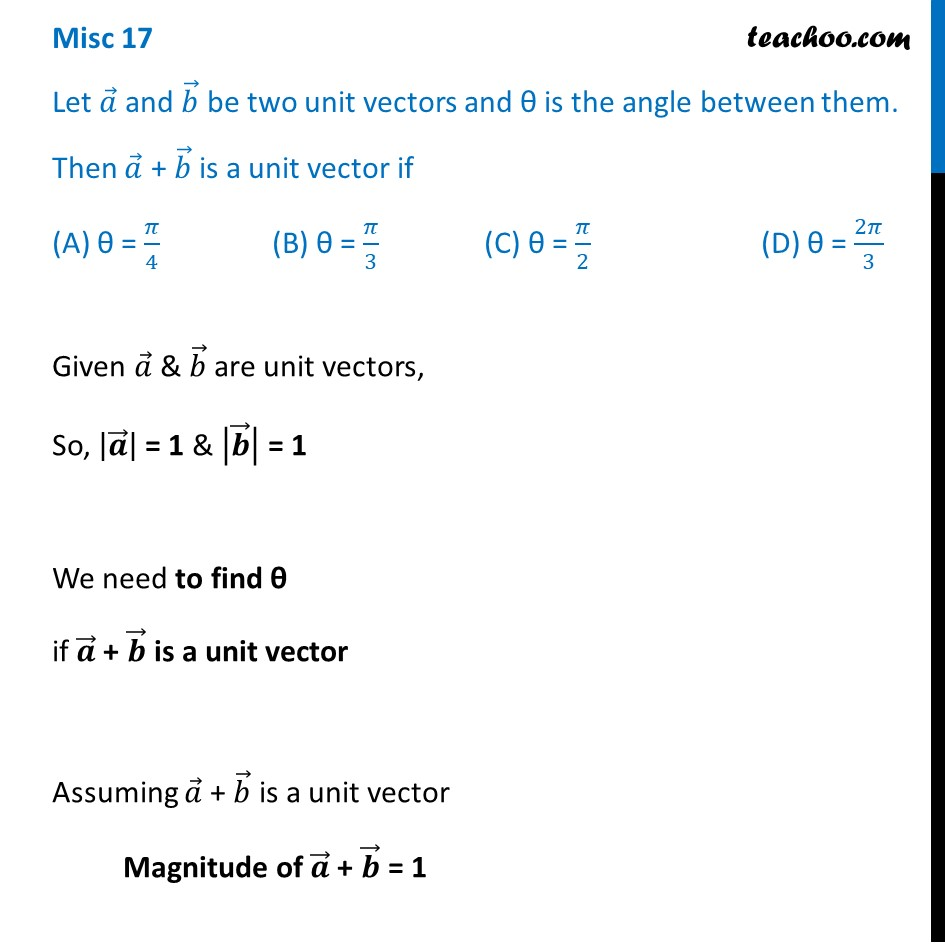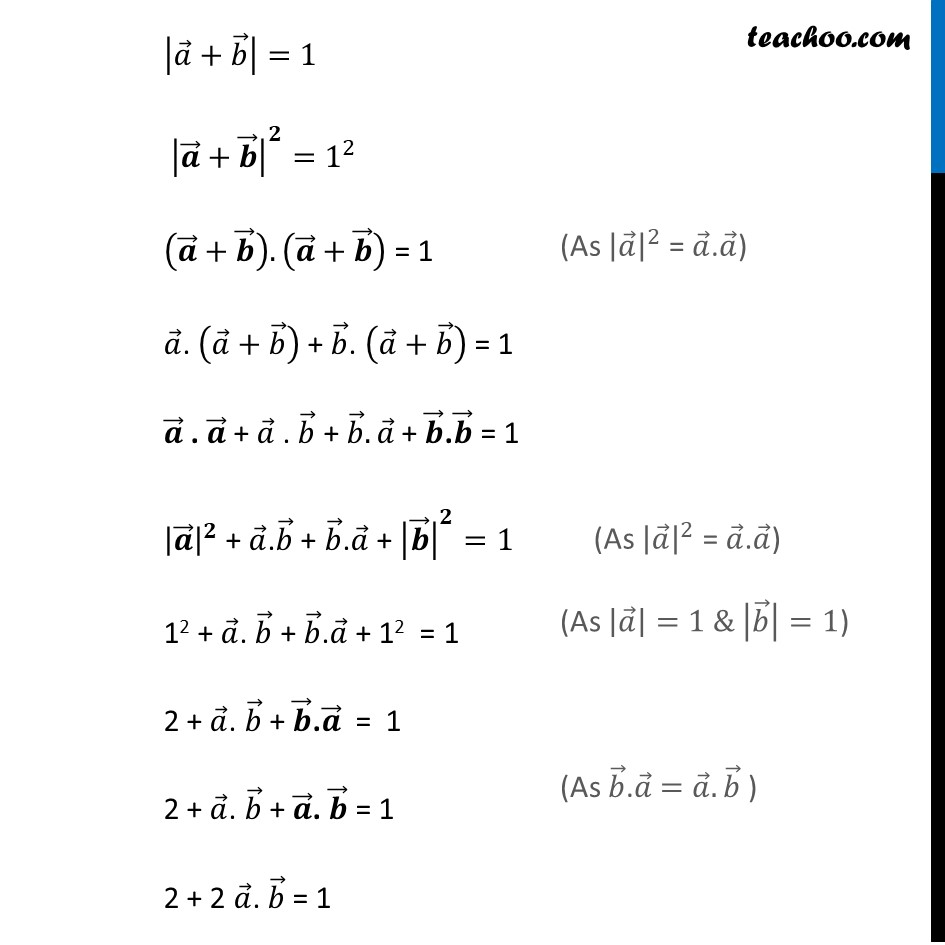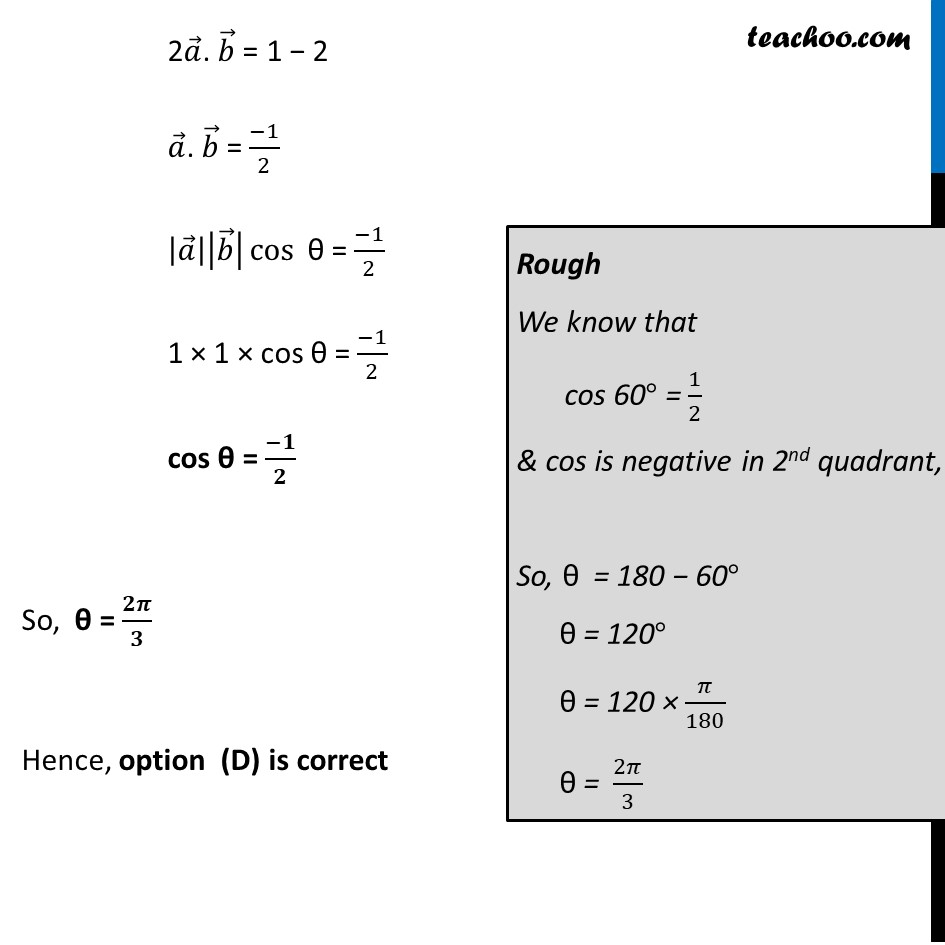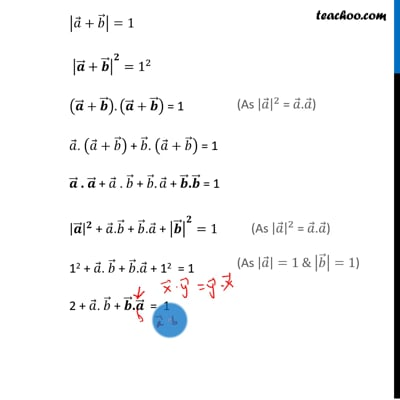Scalar product - Defination

Chapter 10 Class 12 Vector Algebra
Concept wiseThis video is only available for Teachoo black users

Introducing your new favourite teacher - Teachoo Black, at only ₹83 per month

### Transcript

Misc 17 Let 𝑎 ⃗ and 𝑏 ⃗ be two unit vectors and θ is the angle between them. Then 𝑎 ⃗ + 𝑏 ⃗ is a unit vector if (A) θ = 𝜋/4 (B) θ = 𝜋/3 (C) θ = 𝜋/2 (D) θ = 2𝜋/3 Given 𝑎 ⃗ & 𝑏 ⃗ are unit vectors, So, |𝒂 ⃗ | = 1 & |𝒃 ⃗ | = 1 We need to find θ if 𝒂 ⃗ + 𝒃 ⃗ is a unit vector Assuming 𝑎 ⃗ + 𝑏 ⃗ is a unit vector Magnitude of 𝒂 ⃗ + 𝒃 ⃗ = 1 |𝑎 ⃗+𝑏 ⃗ |=1 |𝒂 ⃗+𝒃 ⃗ |^𝟐=1^2 (𝒂 ⃗+𝒃 ⃗ ).(𝒂 ⃗+𝒃 ⃗ ) = 1 𝑎 ⃗. (𝑎 ⃗+𝑏 ⃗ ) + 𝑏 ⃗. (𝑎 ⃗+𝑏 ⃗ ) = 1 𝒂 ⃗ . 𝒂 ⃗ + 𝑎 ⃗ . 𝑏 ⃗ + 𝑏 ⃗.𝑎 ⃗ + 𝒃 ⃗.𝒃 ⃗ = 1 |𝒂 ⃗ |^𝟐 + 𝑎 ⃗.𝑏 ⃗ + 𝑏 ⃗.𝑎 ⃗ + |𝒃 ⃗ |^𝟐=1 12 + 𝑎 ⃗. 𝑏 ⃗ + 𝑏 ⃗.𝑎 ⃗ + 12 = 1 2 + 𝑎 ⃗. 𝑏 ⃗ + 𝒃 ⃗.𝒂 ⃗ = 1 2 + 𝑎 ⃗. 𝑏 ⃗ + 𝒂 ⃗. 𝒃 ⃗ = 1 2 + 2 𝑎 ⃗. 𝑏 ⃗ = 1 2𝑎 ⃗. 𝑏 ⃗ = 1 − 2 𝑎 ⃗. 𝑏 ⃗ = (−1)/2 |𝑎 ⃗ ||𝑏 ⃗ | cos⁡ θ = (−1)/2 1 × 1 × cos θ = (−1)/2 cos θ = (−𝟏)/𝟐 So, θ = 𝟐𝝅/𝟑 Hence, option (D) is correct Rough We know that cos 60° = 1/2 & cos is negative in 2nd quadrant, So, θ = 180 − 60° θ = 120° θ = 120 × 𝜋/180 θ = 2𝜋/3